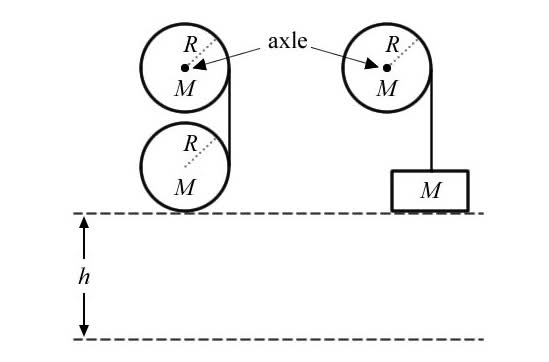# Circular Motion/Torque

• BlameTruth
In summary, the system on the right was used for a previous problem and is therefore useless. The system on the left will have a translational speed of 10 m/s after it has fallen 8 meters.

## Homework Statement

Two identical cylinders of mass M and radius R are mounted on frictionless axles as pictured above. Around each cylinder a massless thread is wound. The hanging thread on the left is wound around a third identical cylinder. A box of mass M is attached to the hanging thread on the right. Both systems are released from rest. After the cylinder has fallen a distance of 8.0 meters, it will have a translational speed of ____ m/s. Use 10 for acceleration due to gravity.On a side note, the system on the right was used for a previous problem.. hence its uselessness.

## Homework Equations

Not sure which of these are relevant:
$$\tau = r x \alpha$$
$$\tau = I \alpha$$
$$KE_t = \frac{1}{2}mv^2$$
$$KE_r = \frac{1}{2}I\omega ^2$$
Gravitational potential energy = $$mgh$$

## The Attempt at a Solution

I tried an approach using Newton's second law and summing the forces that act on the cylinder that is falling.. but attempting that got me down to 1=1 (yay). My friend claims that a conservation of energy approach is the way to go, but I really don't know where to start. If someone could push me in the right direction, that'd be wonderful. This is, apparently, the hardest problem that my AP Physics class will encounter all year (according to the instructor).

Your friend is right about conservation of energy. You should think about the relationship between the translational kinetic energy of the cylinder and its gravitational potential energy. Once you've figured that out, try comparing the situation before and after the cylinder is released.

Oh and welcome to the forum!

Thank you!

So would I be safe in saying that:

$$mgh = \frac{1}{2} mv^2 + \frac{1}{2} I \omega_1 ^2$$

Where omega sub 1 is the angular velocity of the falling cylinder?

Because then I could just go ahead and say that $$v=r\omega_2^2$$

Where omega sub 2 is the angular velocity of the stationary cylinder?

Ok, I might be wrong, but seeing as the question asks only for translational velocity, and the radii of the cylinders are not given, I would go as far as to say that the part of kinetic energy that deals with rotation of the body is unnecessary.

I think you can simply put:

$$mgh = \frac{1}{2} mv^2$$

where h here is the change in height and rearrange to find your answer.

If you use the equation for rotational kinetic energy, the radius ends up getting canceled out. Which leads me to believe that the rotational kinetic is necessary.

I've thought carefully about this because I don't want to send you down the wrong path, and I'm pretty sure that as they ask only for translational energy, you only need worry about the downward movement of the centre of mass of the lower cylinder in a straight line.

But it also has rotational kinetic energy which takes away from the translational. So mgh cannot equal just the translational energy.

Update:

I figured that the angular acceleration of each of the cylinder would be equal, thus the angular velocity once the cylinder has fallen 8m would be equal in each of the cylinders.

So, $$2\omega R = v$$

Then I can solve that for omega and plug that into:

$$mgh = \frac{1}{2} mv^2 + \frac{1}{2}I\omega ^2$$

Any holes in my solution? :/

Sorry, BlameTruth is right and I made a mistake. From what I can tell, your solution should work.